Vibroengineering PROCEDIA

Published: 15 March 2019

# Natural vibration of skew plate on different set of boundary conditions with temperature gradient

Neeraj Lather1
Amit Sharma2
1, 2Department of Mathematics, Amity University Haryana, Gurgaon, India
Corresponding Author:
Amit Sharma
Views 50

#### Abstract

A study is carried out to analyze the natural vibration of non-uniform skew (parallelogram) plate made up of non-homogeneous material on different edge conditions, namely clamped (C), simply supported (S) and free (F), using Rayleigh Ritz method. The thickness and Poisson’s ratio of the plate varies circular in one dimension, while the temperature variation on the plate is viewed to be parabolic in nature. Natural frequencies have been obtained for different combinations of structural parameters involve in the study. The results are compared with the available published results from the open literature.

## 1. Introduction

In order to design an accurate structure, determination of natural frequencies and mode shape are essential. The main aim of the researchers and scientists is to optimize the vibration, for the better performance of the mechanical structures. A significant work about the study of vibrational characteristics of plate has been reported.

A comprehensive study about bending of long, circular, anisotropic plate, plates having lateral loads and forces in the middle on plates and shells has been discussed in . Buckling and bending of thin plates and shells are presented in . Natural vibration of homogeneous, isotropic thin annular and circular plates with variable distributions of parameters by using Green's function and Neumann series has been discussed and presented in [3, 4]. Vibrations of functionally graded material (FGM) rectangular plates with porosities and moving in thermal environment by using von Kármán nonlinear plate theory is discussed in . A detailed study about the vibration of plates (square, rectangle, parallelogram, triangle and circular) is discussed and lots of results are presented for homogenous and uniform plates in . A modeling of non-uniform rectangular plates with various cutouts has been discussed in  to analyze the free vibration of plates with variable thickness. An approximate method using Green function is presented in  to analyze the free vibration of thin and moderately thick rectangular plates with arbitrary variable thickness. Natural vibration of square plate with linear variation in thickness, circular variation in density and exponential variation in Poisson’s ratio along with parabolic temperature variation on clamped edges is studied and first two modes of vibration is evaluated in . Thermal effect on vibration properties of double layered nanoplates at small scale has been studied in . Free vibration of non-homogeneous parallelogram plate with variable thickness under temperature variation is studied and results for first two modes of vibration is presented in [11, 12]. Natural vibration of parallelogram plate with exponential variation in thickness, parabolic variation in density and temperature on mixed edge conditions is studied in . Time period  for frequency parameter of clamped rectangle plate is calculated on various plate parameters using Rayleigh Ritz method.

In this study, authors examine the behavior of vibrational frequency of parallelogram plate with circular variation in thickness and Poisson’s ratio on five edge conditions. The present study also shows the effect of temperature variation on frequency modes. All the obtained results on various variations of structural parameters along with edge conditions are presented in the form of tables. A graphical comparison of results is also given in the form of figures.

## 2. Analysis

Consider a non-uniform and non-homogeneous parallelogram (thin) plate having skew angle $\theta$, length $a$, breadth $b$, thickness $l$, density $\rho$ and Poisson’s ratio $\nu$ referred to skew coordinates $\zeta =x-y\mathrm{t}\mathrm{a}\mathrm{n}\theta$, $\psi =y\mathrm{s}\mathrm{e}\mathrm{c}\theta$.

The kinetic energy ${T}_{s}$ and strain energy ${V}_{s}$ for parallelogram plate is given by:

1
${T}_{s}=\frac{1}{2}{p}^{2}\rho \mathrm{c}\mathrm{o}\mathrm{s}\theta \int \int l{\mathrm{\Phi }}^{2}d\zeta d\psi ,$
2

where $D=E{l}^{3}/\text{12}\left(1-{\nu }^{2}\right)$ and $E$ is known as flexural rigidity and Young’s modulus.

Rayleigh Ritz method requires:

3
$I=\delta \left({V}_{s}-{T}_{s}\right)=0.$

Now introducing circular variation in thickness and Poisson’s ratio as:

4

where ${l}_{0}$ and ${\nu }_{0}$ are the thickness and Poisson’s ratio, respectively at origin. Also and are taper constant and non-homogeneity parameter.

It is also assumed that temperature variation on the plate is parabolic in two dimensions as:

5
$\tau ={\tau }_{0}\left(1-\frac{{\zeta }^{2}}{{a}^{2}}\right)\left(1-\frac{{\psi }^{2}}{{b}^{2}}\right),$

where $\tau$ and ${\tau }_{0}$ is the temperature on the plate at any point and at the origin respectively. The temperature dependence modulus of elasticity is:

6
$E={E}_{0}\left(1-\gamma \tau \right),$

where ${E}_{0}$ is the Young’s modulus at mentioned temperature (i.e., $\tau =$ 0) and $\gamma$ is called slope of variation. Using Eq. (5), Eq. (6) becomes:

7
$E={E}_{0}\left[1-\alpha \left(1-\frac{{\zeta }^{2}}{{a}^{2}}\right)\left(1-\frac{{\psi }^{2}}{{b}^{2}}\right)\right],$

where $\alpha$, $\left(\text{0}\le \alpha <\text{1}\right)$ is called temperature gradient. Using Eqs. (4), (7), Eq. (3) becomes:

8

where ${D}_{0}={E}_{0}{l}_{0}^{3}/12$, $\mathrm{\Lambda }=\left(1-\sqrt{1-\frac{{\zeta }^{2}}{{a}^{2}}}\right)$, ${\lambda }^{2}=\rho {p}^{2}{l}_{0}{a}^{4}/{D}_{0}$ and $\mathrm{Υ}=a/b.$

The two-term deflection function which satisfy all the edge condition can be taken as:

9
$\mathrm{\Phi }\left(\zeta ,\psi \right)=\left[{\left(\frac{\zeta }{a}\right)}^{p}{\left(\frac{\psi }{b}\right)}^{q}{\left(1-\frac{\zeta }{a}\right)}^{r}{\left(1-\frac{\psi }{b}\right)}^{s}\right]\left[\sum _{i=0}^{n}{\mathrm{\Omega }}_{i}{\left\{\left(\frac{\zeta }{a}\right)\left(\frac{\psi }{b}\right)\left(1-\frac{\zeta }{a}\right)\left(1-\frac{\psi }{b}\right)\right\}}^{i}\right],$

where ${\mathrm{\Omega }}_{1}$ and ${\mathrm{\Omega }}_{2}$ represents arbitrary constants and $p$, $q$, $r$. $s$ take values 0, 1 and 2 depending upon the support edge condition i.e., take value 0 for free edge, 1 value for simply supported and 2 value for clamped edge. In order to minimize the functional given in Eq. (8), we require that:

10

After simplifying we get a homogenous system of equations in ${\mathrm{\Omega }}_{i}$ whose non-zero solution gives equation of frequency as:

11
$\left|C-{\lambda }^{2}D\right|=0,$

where $C=\left[{c}_{ij}\right]$ and $D=\left[{d}_{ij}\right]$ are square matrix of order $\left(n+1\right)$, $i=$ 0, 1, 2, ..., $n$ and $j=$ 0, 1, 2, ..., $n.$

## 3. Results and discussion

In order to examine the behavior of natural vibration of non-homogeneous and non-uniform parallelogram plate, a numerical calculation is carried out for different combination of structural parameters on five edge conditions namely, CCCC, CCCS, CSCS, CFCF and CCSF and presented with the help of tables. The value of ${\nu }_{0}$, $\theta$ and $a$/$b$ is taken to be 0.345, 45° and 1.5 respectively.

Table 1 provides the frequency modes corresponding to taper constant $\beta$ for three different set of values of non-homogeneity constant $m$ and thermal gradient $\alpha$ i.e., $m=\alpha =$ 0.2, 0.4, 0.6 on CCCC, CCCS, CSCS, CFCF and CCSF conditions. From Table 1, we analyzed that the frequency of both modes increases with the increasing value of taper constant $\beta$ for all the three set of values of non-homogeneity constant $m$ and thermal gradient $\alpha$ (as mentioned above) on all edge conditions except CFCF condition where frequency modes decreases. On the other aspect when the combined value of non-homogeneity constant $m$ and thermal gradient $\alpha$ increases from 0.2 to 0.6, the frequency modes decreases on all edge conditions except CFCF condition where frequency modes increases. The first mode of frequency is minimum on CFCF condition and maximum on CCCC condition. But the second mode is minimum on CFCF condition and maximum on CCCS condition.

Table 1Tapering parameter β vs vibrational frequency λ for θ= 45° and a/b= 1.5

 BC’s $\beta$ $m=\alpha =$ 0.2 $m=\alpha =$ 0.4 $m=\alpha =$ 0.6 ${\lambda }_{1}$ ${\lambda }_{2}$ ${\lambda }_{1}$ ${\lambda }_{2}$ ${\lambda }_{1}$ ${\lambda }_{2}$ CCCC 0.0 115.41 455.93 109.71 433.63 103.77 410.51 0.4 123.52 484.42 117.63 461.37 111.53 437.56 0.8 132.29 515.19 126.21 491.37 119.92 466.85 CCCS 0.0 95.80 690.46 91.80 680.18 87.69 670.01 0.4 104.11 735.96 99.97 725.19 95.72 714.55 0.8 112.99 784.12 108.68 772.82 104.29 761.69 CSCS 0.0 66.60 533.35 62.89 508.18 59.02 482.11 0.4 71.95 566.25 68.26 540.39 64.43 513.68 0.8 77.88 601.20 74.20 574.59 70.39 547.19 CFCF 0.0 23.01 65.00 24.29 66.90 25.69 68.86 0.4 21.45 58.96 22.85 60.85 24.36 62.82 0.8 19.78 52.21 21.31 54.14 22.97 56.13 CCSF 0.0 29.83 324.28 29.41 319.16 29.03 314.19 0.4 35.92 362.85 35.56 357.38 35.24 352.12 0.8 42.51 403.41 42.20 397.28 41.94 392.00

Table 2Non-homogeneity m vs vibrational frequency λ for θ= 45° and a/b= 1.5

 BC’s $m$ $\beta =\alpha =$ 0.2 $\beta =\alpha =$ 0.4 $\beta =\alpha =$ 0.6 ${\lambda }_{1}$ ${\lambda }_{2}$ ${\lambda }_{1}$ ${\lambda }_{2}$ ${\lambda }_{1}$ ${\lambda }_{2}$ CCCC 0.0 119.87 471.81 118.62 465.27 117.13 457.81 0.4 118.91 468.06 117.63 461.37 116.10 453.75 0.8 118.09 464.78 116.78 458.00 115.24 450.28 CCCS 0.0 100.35 715.83 100.92 731.29 101.37 747.16 0.4 99.44 710.05 99.97 725.19 100.38 740.73 0.8 98.66 704.93 99.17 719.82 99.55 735.10 CSCS 0.0 69.49 551.72 68.87 544.80 68.27 536.82 0.4 68.92 547.45 68.26 540.39 67.62 532.27 0.8 68.44 543.67 67.76 536.50 67.11 528.29 CFCF 0.0 22.41 62.32 23.23 61.39 24.30 60.37 0.4 22.10 61.80 22.85 60.85 23.85 59.81 0.8 21.87 61.34 22.57 60.38 23.53 59.34 CCSF 0.0 32.82 345.36 35.58 361.59 38.50 378.18 0.4 32.81 341.41 35.56 357.38 38.48 373.73 0.8 32.92 338.20 35.70 354.01 38.65 370.19

Table 2 presents the frequency modes corresponding to non-homogeneity constant $m$ for three different set of values of taper constant $\beta$ and thermal gradient $\alpha$ i.e., $\beta =\alpha =$ 0.2, 0.4, 0.6 on CCCC, CCCS, CSCS, CFCF and CCSF conditions. From Table 2, one can easily conclude that vibrational frequency decreases with the increasing value of non-homogeneity constant $m$ for all the three set of values of taper constant $\beta$ and thermal gradient $\alpha$ on all edge conditions except CCSF condition where frequency of first mode increases. On the other hand, frequency modes also decreases when the combined value of taper constant $\beta$ and thermal gradient $\alpha$ increases from 0.2 to 0.6 on all edge conditions except CCCS, CFCF and CCSF condition where frequency modes increases.

Frequency modes corresponding to thermal gradient $\alpha$ for three different set of values of taper constant $\beta$ and non-homogeneity constant $m$ i.e., $\beta =m=$ 0.2, 0.4, 0.6 on CCCC, CCCS, CSCS, CFCF and CCSF conditions is depicted in Table 3. Table 3 enlighten the fact that vibrational frequency decreases with the increasing value of thermal gradient $\alpha$ on all the three value of taper constant $\beta$ and non-homogeneity constant $m$ on all edge conditions and increases with the combined increasing values of taper constant $\beta$ and non-homogeneity constant $m$ on all edge conditions.

Table 3Thermal gradient α vs vibrational frequency λ for θ= 45° and a/b= 1.5

 BC’s $\alpha$ $\beta =m=$ 0.2 $\beta =m=$ 0.4 $\beta =m=$ 0.6 ${\lambda }_{1}$ ${\lambda }_{2}$ ${\lambda }_{1}$ ${\lambda }_{2}$ ${\lambda }_{1}$ ${\lambda }_{2}$ CCCC 0.0 124.47 489.90 128.17 502.71 132.01 515.99 0.4 114.03 448.96 117.63 461.37 121.38 474.25 0.8 102.42 403.93 105.93 415.98 109.58 428.49 CCCS 0.0 103.38 720.51 107.18 740.71 111.11 761.43 0.4 96.24 705.12 99.97 725.19 103.82 745.77 0.8 88.44 689.40 92.11 709.34 95.89 729.79 CSCS 0.0 72.46 572.05 74.89 586.78 77.46 601.92 0.4 65.77 526.03 68.26 540.39 70.84 555.14 0.8 58.31 475.57 60.90 489.62 63.62 504.04 CFCF 0.0 23.01 65.00 24.29 66.90 25.69 68.86 0.4 21.45 58.96 22.85 60.85 24.36 62.82 0.8 19.78 52.21 21.31 54.14 22.97 56.13 CCSF 0.0 33.19 346.68 36.29 364.27 39.57 382.32 0.4 32.41 339.86 35.56 357.38 38.89 375.37 0.8 31.60 332.89 34.81 350.37 38.19 368.29

## 4. Comparison of results

The results (vibrational frequency) of present study is compared with  corresponding to thermal gradient $\alpha$ for fixed value of aspect ratio $a$/$b=$1.5 and skew angle $\theta =$ 30° on CCCC condition and with  corresponding to non-homogeneity constant $m$ and taper constant $\beta$ for fixed value of aspect ratio $a$/$b=$ 1.5 and skew angle $\theta =$ 45° on CCCC condition with the help of figures.

Fig. 1 shows the comparison of frequency modes of present study with  corresponding to thermal gradient $\alpha$ for fixed value of non-homogeneity constant $m$ and taper constant $\beta$ i.e., $m=\beta =$ 0.4 on CCCC edge condition.

Fig. 1Comparison of frequency modes of present study with  corresponding to thermal gradient α on CCCC condition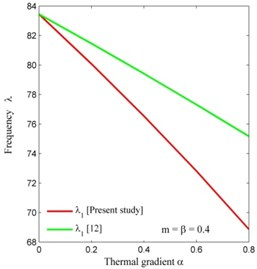a)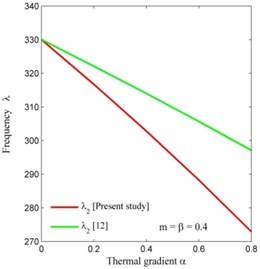b)

From Fig. 1, we conclude that frequency modes of present study is very less when compared to . Frequency modes of present study and  are coincides at $\alpha =$ 0.0.

Figs. 2 and 3 display the comparison of frequency modes of present study with  corresponding to non-homogeneity constant $m$ for fixed value of thermal gradient $\alpha$ and taper constant $\beta$ i.e., $\alpha =\beta =$ 0.2 and taper constant $\beta$ for fixed value of non-homogeneity constant $m$ and thermal gradient $\alpha$ i.e., $m=\alpha =$ 0.0 on CCCC edge conditions. It has been seen that frequencies of both modes are less when compared to . The variation of frequency modes in present study is very less when compared to variation in frequency modes of .

Fig. 2Comparison of frequency modes of present study with  corresponding to non-homogeneity constant m on CCCC condition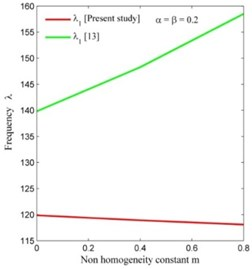a)b)

Fig. 3Comparison of frequency modes of present study with  corresponding to taper constant β on CCCC condition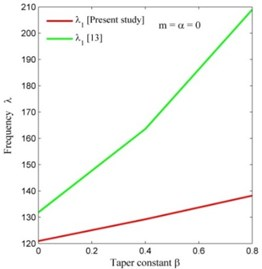a)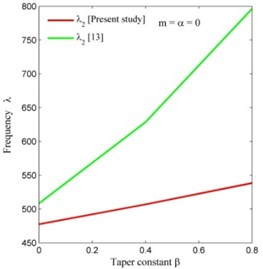b)

## 5. Conclusions

From the above discussions and comparisons of results, we would like to conclude the following points:

1) The frequency modes in case of parabolic temperature variation (present study) are less when compared to linear variation in temperature (as in ) on CCCC condition as shown in Fig. 1.

2) The frequency modes in case of circular variation in non-homogeneity and tapering parameter (present study) are less when compared to parabolic variation in non-homogeneity and exponential variation in tapering parameter (as in ) on CCCC edge conditions as shown in Figs. 2 and 3.

3) The frequencies variation (rate of decrement) in present study is high as compared to .

4) The frequency variation (rate of increment) in present study is less as compared to .

#### Cited by

Thermal Vibration of Rectangle Plate with Two-Dimensional Circular Thickness Effect
Time Period Analysis of Orthotropic Skew Plate with 2-D Circular Thickness and 1-D Circular Density
Soft Computing: Theories and Applications